GFG App
Open AppBrowser
Continue

In Mathematics, there are plethora of shapes and sizes, and Triangles are known to be one of the most important shapes. Triangles in real life can be seen in so many things, for instance, Traffic signals, Front and back view of the pyramids, Bermuda Triangle, etc. Triangles are really significant as it has a base for support and can be used in building bases and trusses.

### Definition

Triangles are three-sided closed polygon formed by the intersection of three lines. It is encountered a lot in everyday life. It is one of the basic shapes of geometry. It has three sides, three angles, and three vertices. There are three types of triangles that we encounter on daily basis- the Equilateral Triangle, Isosceles Triangle, and Scalene Triangle. The figure below shows a triangle ABC with vertices and angles A, B, and C. The sides of this triangle are AB, AC, and BC.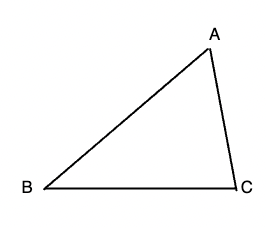Properties of Triangles:

• The sum of all the angles of a Triangle is 180°
• The sum of any two sides of a Triangle will always be greater than the third.
• The difference of any two sides will always be smaller than the third side. (this can be easily deduced by previous property).
• The side which is present opposite to the greatest angle is longest in all three sides.
• The side which is present opposite to the smallest angle is shortest in all three sides. (similar to previous property).
• According to the Exterior Angle property of the triangle, The exterior angle is equal to the sum of the opposite interior angles.

### Types of Triangle

Triangles are classified into two categories: One is based on their side and the other is based on their angle. On the basis of its sides, triangle is divided as Equilateral, Isosceles and scalene triangle. On the basis of its angle, triangles are divided as Acute, Obtuse, and Right and Triangle.

Based on the Side of the Triangle

• Equilateral Triangle

Triangles having all sides and all angles equal are known as equilateral triangle. Since, all the angles are equal, each angle is equal to 60° and the other name of equilateral triangle is Equiangular triangle.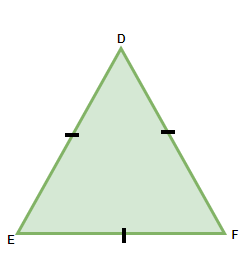• Isosceles Triangle

The triangles having two sides equal and the third one is not equal to the rest two. The angles opposite to the equal sides of the triangle are also equal.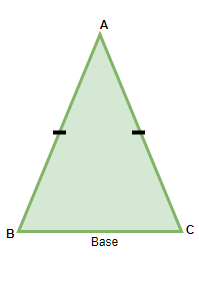• Scalene Triangle

A Scalene triangle is the one which has none of its sides equal to each other and also, none of the angles are equal to each other, but the general properties of the triangle are applied to scalene triangle as well. Hence, the sum of all the interior angles are always equal to 180°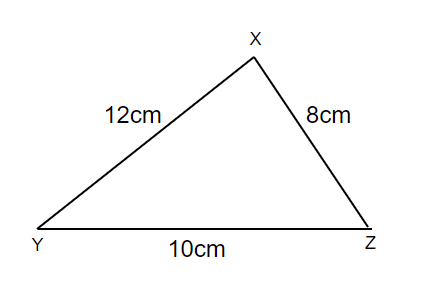Based on the angle of the Triangle

• Acute Angled Triangle

An Acute angled Triangle is the one where all the interior angles of the Triangle is less than 90°. For Instance, an Equilateral Triangle is an acute angled triangle (all angles are less than 90°).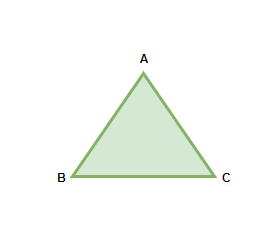An Acute angled triangle

• Right Angled Triangle

A Right Angled Triangle is the one where one of the angles is always equal to 90°. Pythagoras Theorem is derived for Right angled triangles, Which states that the square of the hypotenuse (the longest side) is equal to the sum of the squares of base and perpendicular.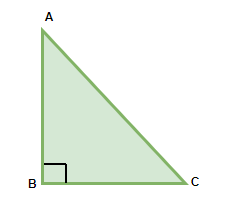A right-angled triangle

•  Obtuse Angled Triangle

An obtuse angled triangle has one of the sides more than 90°, In this case, since one of the three angles is more than 90°, the rest of the two angles is less than 90°.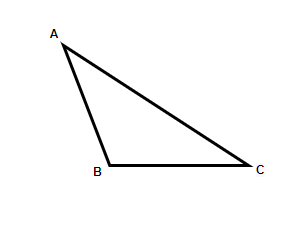An obtuse angled triangle

### Median of a Triangle

Median divides a side into two equal parts. In the context of triangles, a median line from one vertex to the opposite side divides the side into two parts. Every triangle has three medians originating from each vertex. The figure below represents a median from vertex A to the side BC. Notice that the median doesn’t need to be perpendicular, but it definitely needs to bisect the opposite side.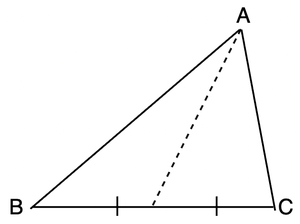Properties of the median of a triangle:

1. The Three medians of any triangle always meet at a single point, no matter what the shape of the triangle. The point at which all three medians meet in a triangle is called the centroid.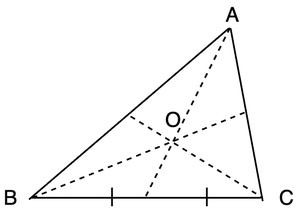2. Median of the triangle divides triangles into two smaller parts which have equal areas.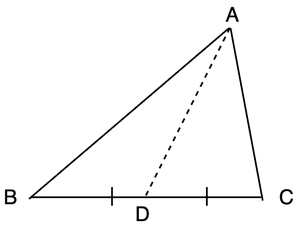3. Let’s say the length of sides of the triangle is “a”, “b” and “c” and the length of medians are l1, l2, and l3

a2 + b2 + c2 = l21 + l22 + l23

### Sum of Angles of Triangle

Polygons with four sides are called quadrilaterals and the sum of their angles is 360°. We know that the sum of angles of triangles is 180°. These properties are the facts and applied for all types of quadrilaterals and Triangles. This is called the Angle Sum Property. The section presents the proof of this property.

Consider the given triangle ABC, in any triangle the sum of all of its angles is 180°. In the given triangle,

∠A + ∠B + ∠C = 180°

Proof: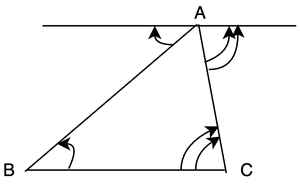In the figure below, draw a line through A which is parallel to BC. Now, these angles are alternate angles which are equal. Thus, from the figure you can see,

∠A + ∠B + ∠C = 180°

### Sample Problems

Question 1: A triangle ABC has ∠A = 60°, ∠B = 60°. Find the angle ∠C.

Solution:

We know that sum of the angles in the triangle is 180°.

∠A + ∠B + ∠C  = 180°

⇒ ∠A + ∠B + ∠C  = 180°

⇒ 60 + 60 + ∠C = 180°

⇒ 120° + ∠C = 180°

⇒ ∠C = 60°

Question 2: Find the missing angle in the given figure.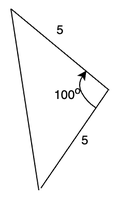Solution:

Let the two missing angles be x and y. Now we can see in the figure that opposite sides of the missing angles are equal in length. That means that the angles must be equal.

So, x = y.

From the angle sum property of the triangle.

x + y + 100° = 180°

⇒ 2x + 100°  = 180°

⇒ 2x = 80°

⇒ x = 40°

Question 3: Find the value of ∠ACD from the given figure.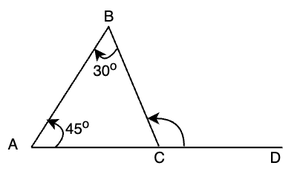Solution:

We know that, ∠ACB and ∠BCD are supplementary angles.

From the angle sum property of the triangle,

∠A + ∠B + ∠C = 180°

⇒ 45° + 30° + ∠C = 180°

⇒ 75° + ∠C = 180°

⇒ ∠C = 105°

∠BCD = 180° – 105°

⇒ ∠BCD = 75°

Question 4: In the given triangle PQR, find the value of ∠PSQ.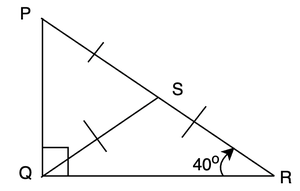Solution:

Considering triangle QSR.

∠SRQ = ∠SQR

⇒ ∠SQR = 40°

Due to angle sum property of the triangle.

∠SQR + ∠SRQ + ∠QSR = 180°

⇒ 40° + 40° + ∠QSR = 180°

⇒ 80° + ∠QSR = 180°

⇒ ∠QSR = 100°

We know,

∠QSR + ∠QSP = 180°

⇒ ∠QSP + 100° = 180°

⇒ ∠QSP = 80°

Question 5: A triangle ABC has angles in the ratio of 1:2:3. Find out the values of all the angles.

Solution:

The interior angles are in the ratio 1:2:3. Thus, they also be written as,

x : 2x: 3x

Using the angle sum property of the triangle.

x + 2x + 3x  = 180°

⇒ 6x = 180°

⇒ x = 30°

Thus, the angles in are,

30°, 60° and 90°.

My Personal Notes arrow_drop_up